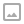## 「POI2011」Tree Rotations-线段树合并

·  ✏️ About  1677 words  ·  ☕ 4 mins read · 👀... views

• 如果 $x==0$ ，表示这个节点非叶子节点，递归地向下读入其左孩子和右孩子的信息；
• 如果 $x!=0$ ，表示这个节点是叶子节点，权值为$x$。

## 链接

Luogu P3521// 数据太弱

LOJ 2163 // 单点时限0.2s 十分适合卡常

## 题解

### 线段树合并

  1 2 3 4 5 6 7 8 9 10 11 12 13  node merge(node u,node v): if u,v 中存在一个节点为空节点: return 另一个节点 // O(1) if u,v 均为叶节点: return 合并u,v 两个叶节点 // O(1) u.leftchild = merge(u.leftchild,v.leftchild) u.rightchild = merge(u.rightchild,v.rightchild) 合并 u.leftchild 和 u.rightchild 的信息为 u // O(1) return u 

## 代码

  1 2 3 4 5 6 7 8 9 10 11 12 13 14 15 16 17 18 19 20 21 22 23 24 25 26 27 28 29 30 31 32 33 34 35 36 37 38 39 40 41 42 43 44 45 46 47 48 49 50 51 52 53 54 55 56 57 58 59 60 61 62 63 64 65 66 67 68 69 70 71 72 73 74 75 76  #include #include using namespace std; #define mid ((l+r)>>1) typedef long long ll; inline char read(){ static const int SIZE = 1024*1024; static char *s,*t,ibuf[SIZE]; if(s == t) t = (s=ibuf) + fread(ibuf,1,SIZE,stdin); return s == t ? -1:(*s++); } template inline void read(T &x){ static bool iosig = 0;static char ch; for(ch = read(),iosig = 0;!isdigit(ch);ch = read()){ if(ch == -1) return; if(ch =='-') iosig = 1; } for(x = 0;isdigit(ch);ch =read()) x = (((x<<2)+x)<<1) + (ch^48); if(iosig) x = -x; } inline ll min(ll a,ll b){ return a > b?b : a; } const int MAXN = 210000; ll ANS = 0,ans1 = 0,ans2 = 0; int n,pos; struct node{ int sumn,ls,rs; }aa[MAXN*30]; int cnt = 0; void update(int &nown,int l,int r){ if(!nown) nown = ++cnt; aa[nown].sumn++; if(l == r) return; if(pos <= mid) update(aa[nown].ls,l,mid); else update(aa[nown].rs,mid+1,r); } void merge(int &lx,int rx){ if(!lx || !rx){lx=lx+rx;return;} aa[lx].sumn += aa[rx].sumn; ans1 += (ll)aa[aa[lx].rs].sumn*aa[aa[rx].ls].sumn; ans2 += (ll)aa[aa[lx].ls].sumn*aa[aa[rx].rs].sumn; merge(aa[lx].ls,aa[rx].ls); merge(aa[lx].rs,aa[rx].rs); } void solve(int &x){ int t,ls,rs;x = 0; read(t); if(!t){ solve(ls),solve(rs); ans1 = ans2 = 0; x = ls;merge(x,rs); ANS += min(ans1,ans2); } else pos = t,update(x,1,n); } signed main(){ read(n); int t = 0; solve(t); printf("%lld\n",ANS); return 0; }WRITTEN BY
cqqqwq
A student in Computer Science.